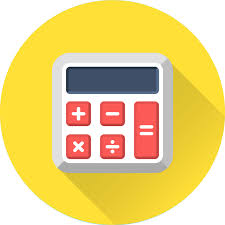# MathsClick the links to find out more:

Maths Y8 Term 1  Measuring the world/IndicesHow do we measure the world? What are more efficient ways of working with number?

Maths Y8 Term 2 Thinking about Ratio and Area and Perimeter - How are different representations of numbers used in mathematics? Why are they needed?

Maths Yr08 T3 Standard Form and Geometric Reasoning - Why is it important to reason in mathematics?

##### year 10

Maths Y10 Term 1 From numbers to letters - What patterns can we find within numbers and calculations?

##### Year 12

Maths Yr12 T1 Algebra and Logarithms

Maths Yr12 T2 Everything Binomial

Maths Yr12 T3 Sequences and Series

##### Year 13

Accordion content